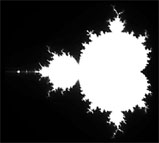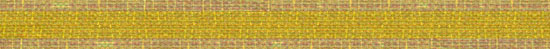Stung Eye

## « [Feb 5] the tyranny of framing our attentionOrdered Chaos Ordered Chaos Revisited [Feb 12] »The above image is a black and white representation of a Mandelbrot set, (the worlds most famous fractal), that I generated using Flash MX 2004. Chances are, you've seen one before. I saw one for the first time in the early 90s and it wasn't until today that I really understood how they were generated. I spent 6 hours today experimenting with this fractal in Flash.

For the lay person:

Imagine the Mandelbrot set as a cage for numbers. Once placed inside the cage, certain numbers can escape very easily, others have to struggle to escape, and some are doomed to remain for eternity. In the above image, these forever 'trapped' numbers are shown in white.

For the geeks:

The Mandelbrot cage wasn't designed for everyday numbers like 16 or 42. No, this cage was designed to trap numbers with imaginary components. The imaginary unit is i, where i is the square root of -1. ( i * i = -1. This is why we call it imaginary, for no real number multiplied by itself can yield a negative result.)

A complex number is one which has both a Real and Imaginary component, a + ib. (Where 'a' is the real component and 'b' is the imaginary component.) Complex numbers are added and multiplied differently than Real Numbers. Let's say I have two complex number, N and G, where N = a + ib and G = c + id:

N + G = (a + c) + i(b + d)
N * G = ac − bd + i (bc + ad)

Our fractal image is built on a grid of complex numbers where the horizontal x axis represents the Real components and the vertical y axis represents the Imaginary components. The Mandelbrot set lives within the region defined by: -3 < x < 2 and -2 < y 2. (Let's call this the Mandelbrot Plane.)

The mathematical cage is defined as follows:

1 - Let C be a complex number within the Mandelbrot Plane.
2 - Let Z = 0.
3 - Find the next value of Z according to this formula: Znew = Zold * Zold + C
4 - Repeat step 3 until Z falls outside of the Mandelbrot Plane (i.e. the number escaped from our cage)

(If Z is truly trapped in our cage we will be able to repeat step 3 infinitely. Therefore, we can assume that our point C belongs inside of the Mandelbrot Set after a fixed number of iterations has been performed. In my flash experiments below, I used a maximum iteration value of 20.)

***

I wrote a collection of Flash Mandelbrot generators today using Actionscript 2.0. I created the first four demos before I realized that I could halve the processing time by assuming that the fractal was symmetrical. Flash was not designed for this kind of number crunching. (Time to learn processing for Java). Please note that images generated by these Flash applets are highly simplified representations of the Mandelbrot set. This is due to both their scale as well as the maximum iteration value I chose. (For a more detailed view visit the Julia and Mandelbrot Set Explorer.)

Note:If you have a slow computer or if you are fairly impatient you can skip the Flash experiments and download an image of their rendered output.

In these first two examples the 'trapped' Mandelbrot numbers are shown in black. The other numbers are displayed in blue according to how fast they escaped from the cage. (The larger and brighter the pixel, the quicker it was able to escape.)

Sideways Mandelbrot with Pixel Scaling and Shading. (Low Rez) - Pre-rendered image

Sideways Mandelbrot with Pixel Scaling and Shading. (High Rez) - Pre-rendered image

These two examples are identical to the previous two, other than the fact that the pixel scaling is now random. (Pseudo-Random)

Sideways Mandelbrot with Random Pixel Scaling. (Low Rez) - Pre-rendered image

Sideways Mandelbrot with Random Pixel Scaling. (High Rez) - Pre-rendered image

Using white circular pixels. (Scaling and brightness are again determined by escape speed.)

Sideways Mandelbrot with Circular Pixel Scaling. (Low Rez) - Pre-rendered image

Sideways Mandelbrot with Circular Pixel Scaling. (High Rez) - Pre-rendered image

A more 'classical' looking Mandelbrot, where the pixel colour represents the escape speed.

Sideways Mandelbrot with Colour Circular Pixel Scaling. - Pre-rendered image

Click here to see some Mandelbrot images I generated using Flash and Photoshop.

***

In my last post I mentioned the Buddhabrot, a sideways Mandelbrot which resembles an image of the Buddha sitting in meditation. This Buddhabrot is generated using the same mathematical 'trap' I described above. However, "If the [complex number] escapes [from the trap] [...], its path is reiterated, exposing its position onto the image surface with each step. In this fashion, areas of dense particle travel appear bright white." In other words, the Buddhabrot is a visualization of the escape path of all the points which don't belong in a Mandelbrot set. Cool!

Comment

--------